NEET  >  31 Year NEET Previous Year Questions: Work, Energy & Power - 2

# 31 Year NEET Previous Year Questions: Work, Energy & Power - 2

Test Description

## 30 Questions MCQ Test Physics Class 11 | 31 Year NEET Previous Year Questions: Work, Energy & Power - 2

31 Year NEET Previous Year Questions: Work, Energy & Power - 2 for NEET 2023 is part of Physics Class 11 preparation. The 31 Year NEET Previous Year Questions: Work, Energy & Power - 2 questions and answers have been prepared according to the NEET exam syllabus.The 31 Year NEET Previous Year Questions: Work, Energy & Power - 2 MCQs are made for NEET 2023 Exam. Find important definitions, questions, notes, meanings, examples, exercises, MCQs and online tests for 31 Year NEET Previous Year Questions: Work, Energy & Power - 2 below.
Solutions of 31 Year NEET Previous Year Questions: Work, Energy & Power - 2 questions in English are available as part of our Physics Class 11 for NEET & 31 Year NEET Previous Year Questions: Work, Energy & Power - 2 solutions in Hindi for Physics Class 11 course. Download more important topics, notes, lectures and mock test series for NEET Exam by signing up for free. Attempt 31 Year NEET Previous Year Questions: Work, Energy & Power - 2 | 30 questions in 60 minutes | Mock test for NEET preparation | Free important questions MCQ to study Physics Class 11 for NEET Exam | Download free PDF with solutions
 1 Crore+ students have signed up on EduRev. Have you?
31 Year NEET Previous Year Questions: Work, Energy & Power - 2 - Question 1

### The co-efficient of restitution e for a perfectly elastic collision is 

Detailed Solution for 31 Year NEET Previous Year Questions: Work, Energy & Power - 2 - Question 1

e = | v1 – v2 |/ | u1 – u2 | which is 1 for a perfectly elastic collision.

31 Year NEET Previous Year Questions: Work, Energy & Power - 2 - Question 2

### A bullet of mass  10g leaves a rifle at an initial velocity of 1000 m/s and strikes the earth at the same level with a velocity of 500 m/s. The work done in joules overcoming the resistance of air will be

Detailed Solution for 31 Year NEET Previous Year Questions: Work, Energy & Power - 2 - Question 2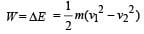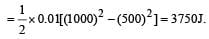31 Year NEET Previous Year Questions: Work, Energy & Power - 2 - Question 3

### How much water, a pump of 2 kW can raise in one minute to a height of 10 m, take g = 10 m/s2?      

Detailed Solution for 31 Year NEET Previous Year Questions: Work, Energy & Power - 2 - Question 3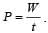Here, P = 2kW = 2000 W..
W = Mgh = M × 10 × 10 = 100 M and t = 60 s.
This gives, M = 1200 kg Its volume = 1200 litre as 1 litre of water contains 1 kg of its mass.

31 Year NEET Previous Year Questions: Work, Energy & Power - 2 - Question 4

Two identical balls A and B moving with velocities +0.5 m/s and –0.3 m/s respectively, collide head on elastically. The velocities of the balls A and B after collision, will be, respectively 

Detailed Solution for 31 Year NEET Previous Year Questions: Work, Energy & Power - 2 - Question 4

When the identical balls collide head-on, their velocities are exchanged.

31 Year NEET Previous Year Questions: Work, Energy & Power - 2 - Question 5

A position dependent force, F = (7 – 2x + 3x2) N acts on a small body of mass 2 kg and displaces it from x = 0 to x = 5 m. Work done in joule is 

Detailed Solution for 31 Year NEET Previous Year Questions: Work, Energy & Power - 2 - Question 5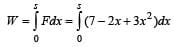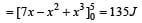31 Year NEET Previous Year Questions: Work, Energy & Power - 2 - Question 6

Two masses of 1g and 9g are moving with equal kinetic energies. The ratio of the magnitudes of their respective linear momenta is 

Detailed Solution for 31 Year NEET Previous Year Questions: Work, Energy & Power - 2 - Question 6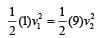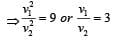Ratio of their linear momenta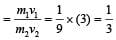31 Year NEET Previous Year Questions: Work, Energy & Power - 2 - Question 7

Consider a car moving along a straight horizantal road with a speed of 72 km/h. If the coefficient of static friction between road and tyres is 0.5, the shortest distance in which the car can be stopped is

Detailed Solution for 31 Year NEET Previous Year Questions: Work, Energy & Power - 2 - Question 7

Force due to friction = kinetic energy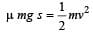[Here, v = 72 km/h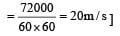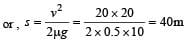31 Year NEET Previous Year Questions: Work, Energy & Power - 2 - Question 8

If the momentum of a body is increased by 50%, then the percentage increase in its kinetic energy is

Detailed Solution for 31 Year NEET Previous Year Questions: Work, Energy & Power - 2 - Question 8

Initial momentum (p1) = p; Final momentum

(p2) = 1.5 p and initial kinetic energy (K1) = K.

Kinetic energy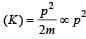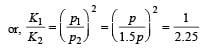or, K2 = 2.25 K.
Therefore, increase in kinetic energy is 2.25 K – K = 1.25 K or 125%.

31 Year NEET Previous Year Questions: Work, Energy & Power - 2 - Question 9

The kinetic energy acquired by a mass (m) in travelling distance (s) starting from rest under the action of a constant force is directly proportional to [1994, 1996]

Detailed Solution for 31 Year NEET Previous Year Questions: Work, Energy & Power - 2 - Question 9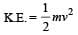Further, v2 = u2 + 2as = 0 + 2ad = 2ad = 2(F/m)d

Hence, K.E. =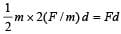or, K.E. acquired = Work done

= F × d = constant.i.e., it is independent of mass m.

31 Year NEET Previous Year Questions: Work, Energy & Power - 2 - Question 10

A body of mass m moving with velocity 3 km/h collides with a body of mass 2 m at rest. Now the coalesced mass starts to move with a velocity 

Detailed Solution for 31 Year NEET Previous Year Questions: Work, Energy & Power - 2 - Question 10

Applying law of conservation of momentum,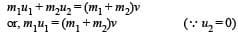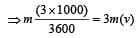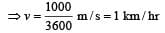31 Year NEET Previous Year Questions: Work, Energy & Power - 2 - Question 11

Two bodies of masses m and 4 m ar e moving with equal K.E. The ratio of their linear momenta is

Detailed Solution for 31 Year NEET Previous Year Questions: Work, Energy & Power - 2 - Question 11

m1 = m, m2 = 4 m K . E1 = K . E2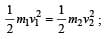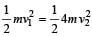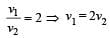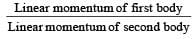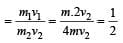31 Year NEET Previous Year Questions: Work, Energy & Power - 2 - Question 12

A molecule of mass m of an ideal gas collides with the wall of a vessel with a velocity v and returns back with the same velocity. The change in linear momentum of molecule is 

Detailed Solution for 31 Year NEET Previous Year Questions: Work, Energy & Power - 2 - Question 12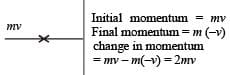31 Year NEET Previous Year Questions: Work, Energy & Power - 2 - Question 13

A metal ball of mass 2 kg moving with a velocity of 36 km/h has a head on collision with a stationary ball of mass 3 kg. If after the collision, the two balls move together, the loss in kinetic energy due to collision is 

Detailed Solution for 31 Year NEET Previous Year Questions: Work, Energy & Power - 2 - Question 13

Applying conservation of momen tum,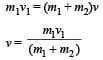Here, v1 = 36 km/hr = 10 m/s m1 = 2 kg, m2 = 3 kg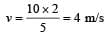K.E. (initial) =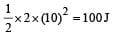K.E. (Final) =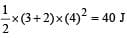Loss in K.E. = 100 – 40 = 60 J

31 Year NEET Previous Year Questions: Work, Energy & Power - 2 - Question 14

Two equal masses m1 and m2 moving along the same straight line with velocities + 3 m/s and – 5m/s respectively, collide elastically.Their velocities after the collision will be respectively. 

Detailed Solution for 31 Year NEET Previous Year Questions: Work, Energy & Power - 2 - Question 14

In elastic collision, the velocities get in ter changed if the colliding objects have equal masses.

31 Year NEET Previous Year Questions: Work, Energy & Power - 2 - Question 15

A force acts on a 30 gm particle in such a way that the position of the particle as a function of time is given by x = 3t – 4t2 + t3, where x is in metres and t is in seconds. The work done during the first 4 seconds is 

Detailed Solution for 31 Year NEET Previous Year Questions: Work, Energy & Power - 2 - Question 15

x = 3t –4t2 + t3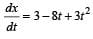Acceleration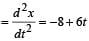Acceleration after 4sec = –8 + 6 × 4 = 16 ms–2
Displacement in 4sec = 3 ×4 – 4 × 42 + 43 = 12 m

∴  Work = Force × displacement = Mass × acc. × disp. = 3 × 10–3 × 16 × 12 = 576 mJ

31 Year NEET Previous Year Questions: Work, Energy & Power - 2 - Question 16

A rubber ball is dropped from a height of 5m on a plane, where the acceleration due to gravity is not shown. On bouncing it rises to 1.8 m. The ball loses its velocity on bouncing by a factor of 

Detailed Solution for 31 Year NEET Previous Year Questions: Work, Energy & Power - 2 - Question 16

Accordin g to principle of conservation of energy Loss in potential energy = Gain in kinetic energy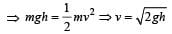If  h1 and h2 are initial and final heights, then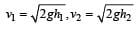Loss in velocity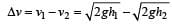∴  Fractional loss in velocity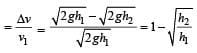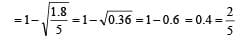31 Year NEET Previous Year Questions: Work, Energy & Power - 2 - Question 17

Two bodies with kinetic energies in the r atio 4 : 1 are moving with equal linear momentum. The ratio of their masses is 

Detailed Solution for 31 Year NEET Previous Year Questions: Work, Energy & Power - 2 - Question 17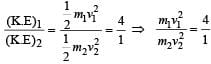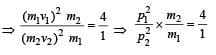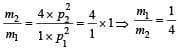[Given: p1=p2]

31 Year NEET Previous Year Questions: Work, Energy & Power - 2 - Question 18

A 3 kg ball strikes a heavy rigid wall with a speed of 10 m/s at an angle of 60º. It gets reflected with the same speed and angle as shown here. If the ball is in contact with the wall for 0.20s, what is the average force exerted on the ball by the wall?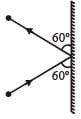Detailed Solution for 31 Year NEET Previous Year Questions: Work, Energy & Power - 2 - Question 18

Change in momentum along the wall = mv cos60º – mv cos 60º = 0
Change in momentum perpendicular to the wall
= mv sin60º – (– mv sin60º) = 2mv sin60º

∴ Applied force =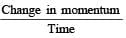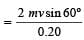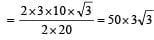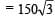newton

31 Year NEET Previous Year Questions: Work, Energy & Power - 2 - Question 19

A bomb of mass 1 kg is thr own vertically upwards with a speed of 100 m/s. After 5 seconds it explodes into two fragments. One fragment of mass 400 gm is found to go down with a speed of 25 m/s. What will happen to the second fragment just after the explosion? (g = 10 m/s2) 

Detailed Solution for 31 Year NEET Previous Year Questions: Work, Energy & Power - 2 - Question 19

Speed of bomb after 5 second, v = u – gt  = 100 –10×5 = 50m/s

Momentum of 400 g fragment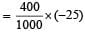[downward]

Momentum of 600g fragment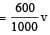Momentum of bomb = 1 × 50 = 50

From conservation of momentum Total momentum before splitting = total momentum after splitting.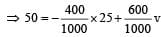⇒ v = 100 m/s [upward]

31 Year NEET Previous Year Questions: Work, Energy & Power - 2 - Question 20

In a simple pendulum of length l the bob is pulled aside from its equilibrium position through an angle θ and then released. The bob passes through the equilibrium position with speed.     

Detailed Solution for 31 Year NEET Previous Year Questions: Work, Energy & Power - 2 - Question 20

If l is length of pendulum and θ be angular amplitude then height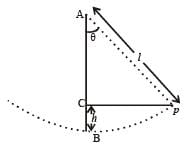h = AB AC = l – l cosθ = l(1 –  cosθ)
At extreme position, potential energy is maximum and kinetic energy is zero; At mean (equilibrium) position potential energy is zero and kinetic energy is maximum, so from principle of conservation of energy. (KE + PE ) at P = (KE + PE) at B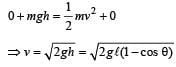31 Year NEET Previous Year Questions: Work, Energy & Power - 2 - Question 21

A force of 250 N is required to lift a 75 kg mass through a pulley system. In order to lift the mass through 3 m, the rope has to be pulled through 12m. Th e efficiency of system is 

Detailed Solution for 31 Year NEET Previous Year Questions: Work, Energy & Power - 2 - Question 21

Efficiency =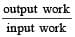i.e. Efficiency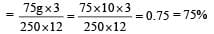31 Year NEET Previous Year Questions: Work, Energy & Power - 2 - Question 22

If the kinetic energy of a particle is increased by 300%, the momentum of the particle will increase by

Detailed Solution for 31 Year NEET Previous Year Questions: Work, Energy & Power - 2 - Question 22

New K.E.,  E' = 4E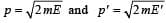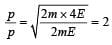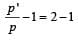[on substrating 1 in both sides.]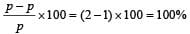31 Year NEET Previous Year Questions: Work, Energy & Power - 2 - Question 23

When a long sprin g is stretched by 2 cm, its potential energy is U. If the spring is stretched by 10 cm, the potential energy stored in it will be

Detailed Solution for 31 Year NEET Previous Year Questions: Work, Energy & Power - 2 - Question 23

If k be the spring constant, then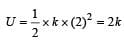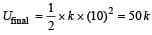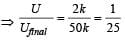⇒ U final = 25U

31 Year NEET Previous Year Questions: Work, Energy & Power - 2 - Question 24

A stationary particle explodes into two particles of masses m1 and m2 which move in opposite directions with velocities v1 and v2. The ratio of their kinetic energies E1/E2 is 

Detailed Solution for 31 Year NEET Previous Year Questions: Work, Energy & Power - 2 - Question 24

From conservation law of momentum, before collision and after collision linear momentum (p) will be same. That is, initial momentum = final momentum.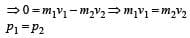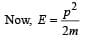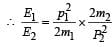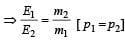31 Year NEET Previous Year Questions: Work, Energy & Power - 2 - Question 25

A mass of 0.5 kg moving with a speed of 1.5 m/ s on a horizontal smooth surface, collides with a nearly weightless spring of force constant k = 50 N/m. The  maximum compression of the spring would be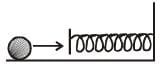Detailed Solution for 31 Year NEET Previous Year Questions: Work, Energy & Power - 2 - Question 25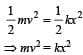or 0.5 × (1.5)2 = 50×x2

∴  x = 0.15 m

31 Year NEET Previous Year Questions: Work, Energy & Power - 2 - Question 26

A particle of mass m1 is moving with a velocity v1 and another particle of mass m2 is moving with a velocity v2. Both  of them have the same momentum but their different kinetic energies are E1 and E2 respectively. If m1 > m2 then 

Detailed Solution for 31 Year NEET Previous Year Questions: Work, Energy & Power - 2 - Question 26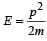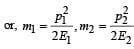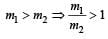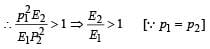or, E2 > E1

31 Year NEET Previous Year Questions: Work, Energy & Power - 2 - Question 27

A ball of mass 2 kg and another of mass 4 kg are dropped together from a 60 feet tall building.After a fall of 30 feet each towards earth, their respective kinetic energies will be in the ratio of 

Detailed Solution for 31 Year NEET Previous Year Questions: Work, Energy & Power - 2 - Question 27

Since height is same for both balls, their velocities on reaching the ground will be same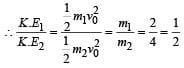31 Year NEET Previous Year Questions: Work, Energy & Power - 2 - Question 28

A force F acting on an object varies with distance x as shown here. The force is in N and x in m. The work done by the force in moving the object fr om x = 0 to x = 6 m is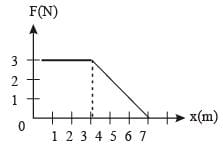Detailed Solution for 31 Year NEET Previous Year Questions: Work, Energy & Power - 2 - Question 28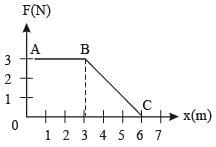Work done  = area under F-x graph = area of trapezium OABC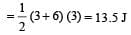31 Year NEET Previous Year Questions: Work, Energy & Power - 2 - Question 29

A bomb of mass 30 kg at rest explodes into two pieces of masses 18 kg and 12 kg. The velocity of 18 kg mass is 6 ms–1. The kinetic energy of the other mass is 

Detailed Solution for 31 Year NEET Previous Year Questions: Work, Energy & Power - 2 - Question 29

From conservation of linear momentum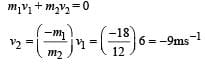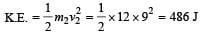31 Year NEET Previous Year Questions: Work, Energy & Power - 2 - Question 30

A body of  mass 3 kg is under a constant force which causes a displacements in metres in it, given by the relation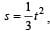, where t is in seconds.
Work done by the force in 2 seconds is 

Detailed Solution for 31 Year NEET Previous Year Questions: Work, Energy & Power - 2 - Question 30

Acceleration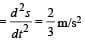Force acting on the body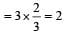newton

Displacement in 2 secs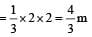Work done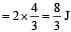## Physics Class 11

127 videos|464 docs|210 tests
 Use Code STAYHOME200 and get INR 200 additional OFF Use Coupon Code
Information about 31 Year NEET Previous Year Questions: Work, Energy & Power - 2 Page
In this test you can find the Exam questions for 31 Year NEET Previous Year Questions: Work, Energy & Power - 2 solved & explained in the simplest way possible. Besides giving Questions and answers for 31 Year NEET Previous Year Questions: Work, Energy & Power - 2, EduRev gives you an ample number of Online tests for practice

## Physics Class 11

127 videos|464 docs|210 tests

### How to Prepare for NEET

Read our guide to prepare for NEET which is created by Toppers & the best Teachers